Function Repository Resource:

# PulmonaryDiffusingCapacity

Calculate the rate of transfer of CO gas from air in the lungs to the blood

Contributed by: Claudio Lobo Chaib Filho
 ResourceFunction["PulmonaryDiffusingCapacity"][gender,age,height] gives the pulmonary diffusing capacity of carbon monoxide for a specific gender,age and height. ResourceFunction["PulmonaryDiffusingCapacity"][gender, age, height, out] returns the output specified by out.

## Details and Options

ResourceFunction["PulmonaryDiffusingCapacity"] measures the transfer of CO gas from air in the lungs to the red blood cells in lung blood vessels. The default output unit is milliliters of CO per minutes per millimeters of Mercury.
Gender (gender) can be given as an Entity or String, e.g. Entity["Gender","Male"] or "Female".
The function can accept ages in the range of 18 to 65 years. If given as Quantity, any time unit is accepted. When not specified, the unit of time is taken to be years.
The height can be from 92 to 244 centimeters. If given as Quantity, any height unit is accepted. When not specified, the height unit is assumed to be centimeters.
The output specification out accepts the following values:
 "DiffusingCapacity" (DLCO) diffusing capacity for carbon monoxide "Plot" generate a plot for comparison "PropertyAssociation" include all output in an Association
A plot is generated as a curve in which the x-axis is age (in years) and the y-axis is "DiffusingCapacity" (in milliliters of CO per minute per millimeter of Hg) for a given gender and height.

## Examples

### Basic Examples (1)

Get the value of the pulmonary diffusing capacity of carbon monoxide for a specific male:

 In:=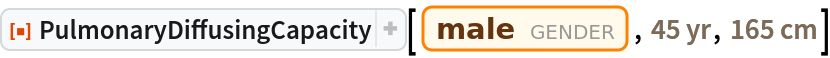Out=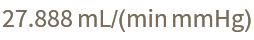### Scope (5)

If values without Quantity are used, the age is interpreted as "Years" and the height as "Centimeters":

 In:=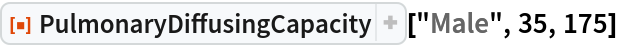Out=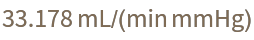In:=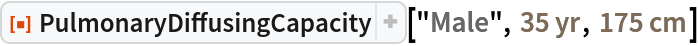Out=Other time and length units are accepted as well:

 In:=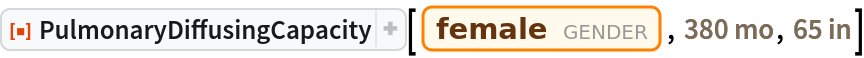Out=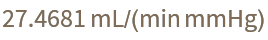Create a plot using the "Plot" output. The x-axis is age (given in years) and the y-axis is "DiffusingCapacity" (DLCO) (in milliliters of CO per minute per millimeter of Hg) for a given gender and height:

 In:=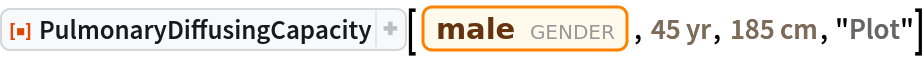Out=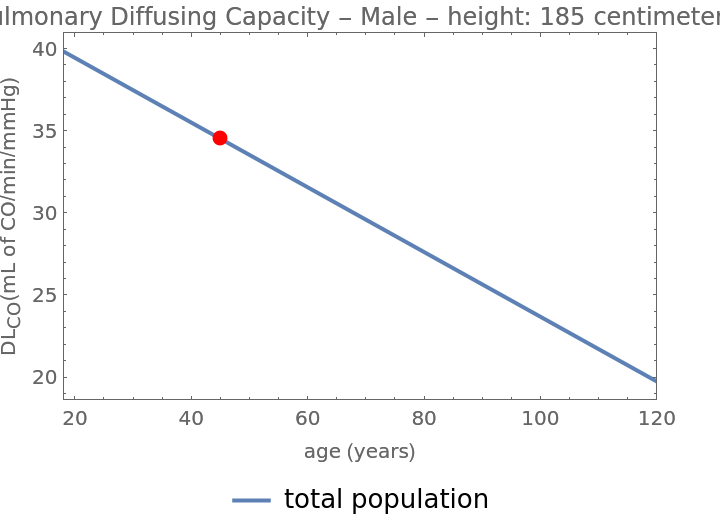Specify "PropertyAssociation" as the output:

 In:=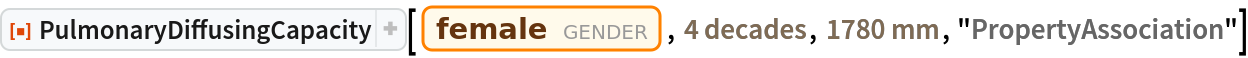Out=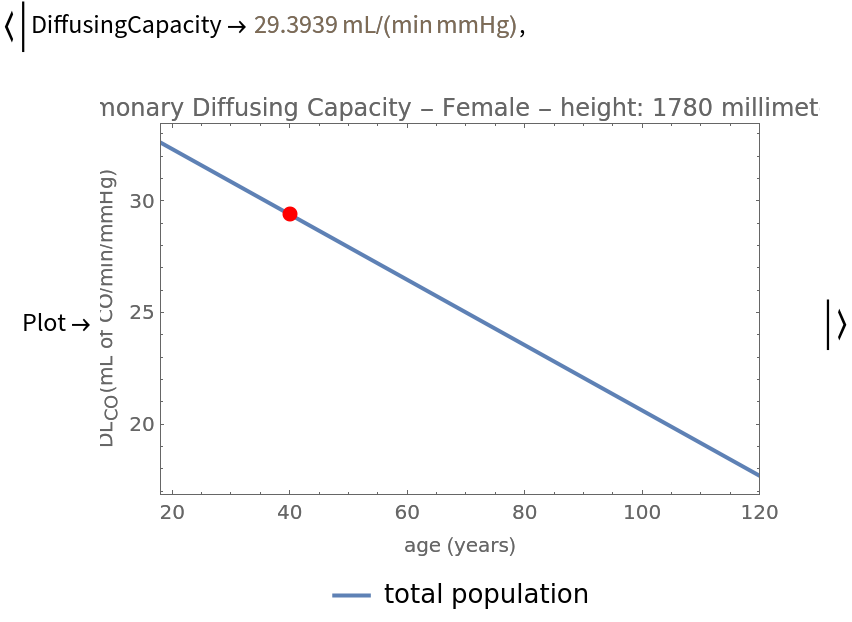The default output is "DiffusingCapacity":

 In:=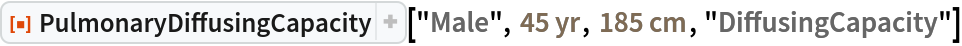Out=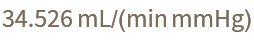In:=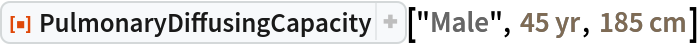Out=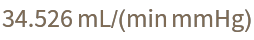### Possible Issues (1)

The x-axis of the plot is given in years, regardless of the inputs:

 In:=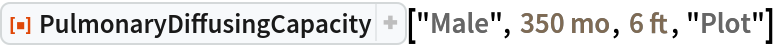Out=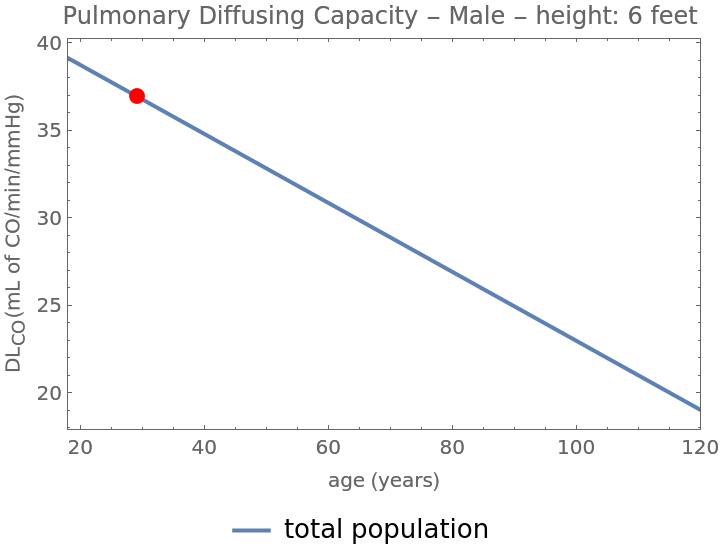## Publisher

Claudio Lobo Chaib Filho

## Version History

• 1.0.0 – 12 November 2020